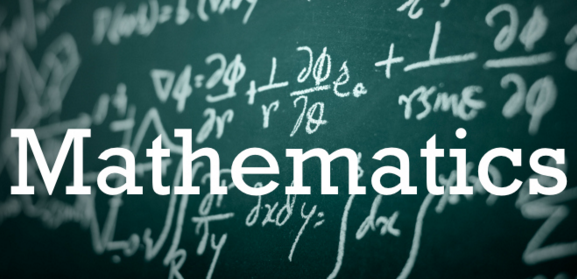# Combination + Permutation = Combutation

Probability Level 4Suppose C be the number of combinations and P be the number of permutations of four alphabets taken from the word MATHEMATICS. Find the value of (C+P).

×